Linear regression is a great start to the journey of machine learning, given that it is a pretty straightforward problem and can be solved by popular modules such as the scikit-learn package. In this article, we shall discuss a line-by-line approach on we implement linear regression using TensorFlow.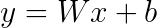linear regression equation

Looking at the equation of linear regression above, we begin by constructing a graph that learns the gradient of the slope (W) and bias (b) through multiple iterations. In each iteration, we aim to close up the gap (loss) by comparing input y to the predicted y. This means to say, we want to modify and b such that inputs of x will give us the y we want. Solving the linear regression is also known as finding the line of best fit or trend line.

#### Generating Dataset

[line 1, 2, 3]

```import numpy as np
import tensorflow as tf
import matplotlib.pyplot as plt
```

In this article, we will use some of the popular modules such as numpytensorflow and matplotlib.pyplot. Let’s import them.

[line 6, 7]

```x_batch = np.linspace(0, 2, 100)
y_batch = 1.5 * x_batch + np.random.randn(*x_batch.shape) * 0.2 + 0.5
```

To begin, we start by generating our dataset, namely x and y. You can think of each value in x and y as points on the graph. In line 6, we want numpy to generate 100 points with value between 0 and 2, spread evenly. The result is a numpy array stored in `x_batch`. Similarly, we also want to randomly generate y such that it has a gradient of 1.5 (W) and some form of randomness using `np.random.randn()`. To make things interesting, we set y-intercept b to 0.5.

[line 8] `return x_batch, y_batch`

We return both numpy arrays `x_batch` and `y_batch`.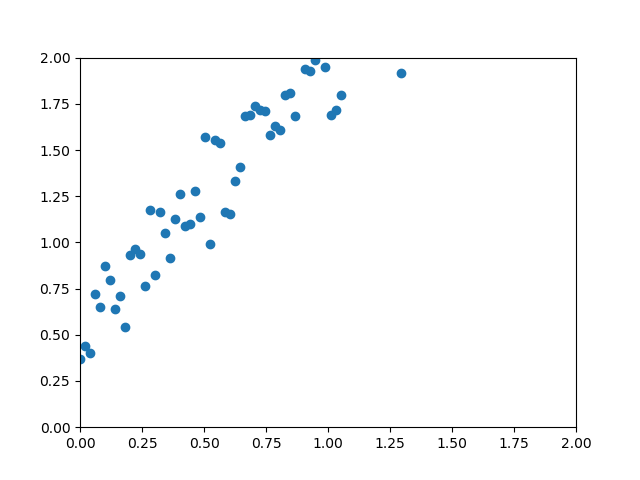plt.scatter(x_batch, y_batch) — this is our starting point

This is how the plot looks like with `generate_dataset()`. Notice that visually, the points form a trend line starting from the bottom left to the top right but not cutting through the origin (0, 0).

#### Constructing the Graph

[line 2 and 3]

```x = tf.placeholder(tf.float32, shape=(None, ), name='x')
y = tf.placeholder(tf.float32, shape=(None, ), name='y')
```

Next, we construct the TensorFlow graph that helps us compute and b. This is done in the function `linear_regression()`. In our formula `y = Wx + b`, the x and y are nodes represented as TensorFlow’s placeholder. Declaring x and yas placeholders mean that we need to pass in values at a later time — we will revisit this in the following section. Note that we are now merely constructing the graph and not running it (TensorFlow has lazy evaluation).

In the first argument of `tf.placeholder`, we define the data type as float32 — a common data type in placeholder. The second argument is the shape of the placeholder set to `None` as we want it to be determined during training time. The third argument lets us set the name for the placeholder.

tf.placeholder – A placeholder is simply a variable that we will assign data to at a later date. It allows us to create our operations and build our computation graph, without needing the data. In TensorFlow terminology, we then feed data into the graph through these placeholders.

https://learningtensorflow.com/lesson4/

[line 5]`with tf.variable_scope(‘lreg’) as scope:`

This line defines the variable scope for our variables in line 6 and 7. In short, Variable scope allows naming of variable in a hierarchy way to avoid name clashes. To elaborate, it is a mechanism in TensorFlow that allows variables to be shared in different parts of the graph without passing references to the variable around. Note that even though we do not reuse variables here, it is a good practice to name them appropriately.

```with tf.name_scope("foo"):
with tf.variable_scope("var_scope"):
v = tf.get_variable("var", )
with tf.name_scope("bar"):
with tf.variable_scope("var_scope", reuse=True):
v1 = tf.get_variable("var", )
assert v1 == v
print(v.name)   # var_scope/var:0
print(v1.name)  # var_scope/var:0
```

In the code above, we see that the variable (“var”) is reused and asserted to be true. To use the same variable, just call `tf.get_variable(“var”, )`.

[line 6]`w = tf.Variable(np.random.normal(), name=’W’)`

Different from a placeholder, W is defined as a `tf.Variable` where the value changes as we train the model, each time ending with lower loss. In line 10, we will explain what “loss” means. For now, we set the variable using `np.random.normal()` so that it draw a sample from the normal (Gaussian) distribution.

tf.Variable — A variable maintains state in the graph across calls to `run()`. You add a variable to the graph by constructing an instance of the class `Variable`.
The `Variable()` constructor requires an initial value for the variable, which can be a `Tensor` of any type and shape. The initial value defines the type and shape of the variable. After construction, the type and shape of the variable are fixed. The value can be changed using one of the assign methods.

https://www.tensorflow.org/api_docs/python/tf/Variable

Note that even though the variable is now defined, it has to be explicitly initialised before you can run operation using that value. This a feature of lazy evaluation and we will do the actual initialisation later.

What W is really doing here is to find the gradient of our line of best fit. Previously, we generated the dataset using a gradient of 1.5 so we should expect the trained W to be close to this number. Selecting the starting number for W is somewhat important — imagine the work we save if we could “randomly” select 1.5, job’s done isn’t it? About right…

Since we are on this topic of searching for the optimal gradient in linear regression, I need to point out that our loss function will always result in one minimum loss value regardless of where we initialise W. This is due to the convexity of our loss function, W and b when we plot them in a chart like this. In other words, this bowl shape figure allows us to identify the lowest point, regardless of where we start.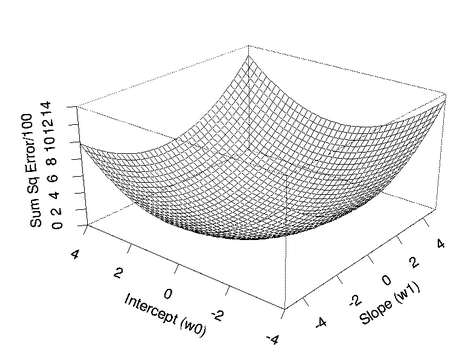One global minimum

However, this is not the case for more complex problems where there are multiple local minima like the one shown below. Choosing a bad number to initialise your variables could result in your gradient search being stuck at one of the local minima. This prevents you from reaching the global minimum which has a lower loss.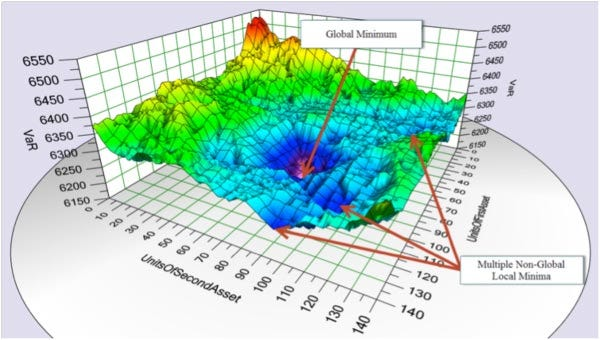Multiple local minima with one global minimum

Researchers have come up with alternate methods of initialisation such as Xavier initialisation in attempt to avoid this problem. If you feel like using it, feel free to do so with:

`tf.get_variable(…, initializer=tf.contrib.layers.xavier_initializer())`.

[line 7] `b = tf.Variable(np.random.normal(), name=’b’)`

Other than W, we also want to train our bias b. Without b, our line of best fit will always cut through the origin and not learn the y-intercept. Remember the 0.5? We need to learn that as well.

[line 9]`y_pred = tf.add(tf.multiply(w, x), b)`

After defining xy and W individually, we are now ready to put them together. To implement the formula `y = Wx + b`, we start off by multiplying `w` and `x` using `tf.multiply` before adding the variable `b`using `tf.add`. This will perform an element-wise multiplication and then addition which results in a tensor `y_pred``y_pred` represents the predicted y value and as you might be suspecting, the predicted y will be terrible at first and is far off from the generated y. Similar to a placeholder or variable, you are free to put a name to it.

[line 11]`loss = tf.reduce_mean(tf.square(y_pred — y))`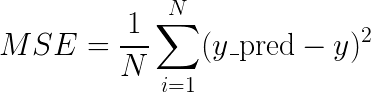Mean Squared Error (MSE)

After calculating `y_pred`, we want to know how far the predicted y is away from our generated y. To do this, we need to design a method to calculate the “gap”. This design is known as the loss function. Here, we selected the Mean Squared Error (MSE) a.k.a. L2 loss function as our “scoring mechanism”. There are other popular loss functions but we are not covering them.

To understand our implementation of MSE, we first find the difference between each of the 100 points for `y_pred` and `y` using `y_pred — y`. Next, we amplify their difference by squaring them (`tf.square`), thereby making the difference (a lot) larger. Ouch! 😝

With a vector size of 100, we now have a problem — how can we know if these 100 values represent a good score or not? Usually a score is a single number that determines how well you perform (just like your exams). So to get to a single value, we make use of `tf.reduce_mean` to find the mean of all the 100 values and set it as our `loss`.

[line 13]`return x, y, y_pred, loss`

Last but not least, we return all the 4 values after constructing them.

#### Computing the Graph

With `generate_dataset()` and `linear_regression()`, we are now ready to run the program and begin finding our optimal gradient W and bias b!

[line 2, 3]

```x_batch, y_batch = generate_dataset()
x, y, y_pred, loss = linear_regression()
```

In this `run()` function, we start off by calling `generate_dataset()` and `linear_regression()` to get `x_batch``y_batch``x``y``y_pred` and `loss`. Scroll up to see explanation for these two functions.

[line 5, 6]

```optimizer = tf.train.GradientDescentOptimizer(0.1)
train_op = optimizer.minimize(loss)
```

Then, we define the optimiser and ask it to minimise the loss in the graph. There are several optimisers to choose from and we conveniently selected the Gradient Descent algorithm and set the learning rate to 0.1.

We will not dive into the world of optimisation algorithms but in short, the job of an optimiser is to minimise (or maximise) your loss (objective) function. It does so by updating the trainable variables (W and b) in the direction of the optimal solution everytime it runs.

Calling the minimize function computes the gradients and applying them to the variables — this is the behaviour by default and you are free to change it using the argument `var_list`.

[line 8] `with tf.Session() as session:`

In the earlier part where we construct the graph, we said that TensorFlow uses lazy evaluation. This really means that the graph is only computed when a session starts. Here, we name the session object as `session`.

[line 9] `session.run(tf.global_variables_initializer())`

Then we kickstart our first session by initialising all the values we ask the variables to hold. Due to lazy evaluation, variables e.g. W (`w = tf.Variable(np.random.normal(), name=’W’)`) are not initialised when the graph is first constructed, until we run this line. See this for further explanation.

[line 10] `feed_dict = {x: x_batch, y: y_batch}`

Next, we need to come up with feed_dict which is essentially an argument for `session.run()``feed_dict` is a dictionary with its key being a `tf.Tensor``tf.placeholder` or `tf.SparseTensor`. The `feed_dict` argument allows the caller to override the value of the tensors (scalar, string, list, numpy array or tf.placeholder e.g. x and y) in the graph.

In this line, the x and y are the placeholders and x_batch and y_batch are the values generated, ready to fill up the placeholders during `session.run()`.

[line 12] `for i in range(30):`

After initialising the variables and preparing values for placeholders using `feed_dict`, we now come to the core of the script which is to define how many times we want to “adjust” / “train” the weight (W) and bias (b). The number of times we go through the training data (x and y) in one full cycle is also known as epoch / training step. One full cycle is also defined as a one feedforward and one backpropagation.

During feedforward, we pass in the value of xw and b to get the predicted y. This computes the loss which is represented by a number. As the objective of this graph is to minimise the loss, the optimiser will then perform a backpropagation to “adjust” the trainable variables (W and b) so that the next time we perform the feedforward (in another epoch), the loss will be lowered.

We do this forward and backward cycle for 30 times. Note that 30 is a hyperparameter and you are free to change it. Also note that more epochs = longer training time.

[line 13]

```session.run(train_op, feed_dict)
```

Now we are ready to run our first epoch by calling`session.run()` with fetches and `feed_dict`. Over here, `session.run()` evaluates every tensor in fetches (`train_op`) and substitutes the values in `feed_dict` for the corresponding input values.

`fetches`: A single graph element, a list of graph elements, or a dictionary whose values are graph elements or lists of graph elements (see documentation for `run`).

What happens behind the scene when the `run()` method is called by `session`object is that your code will run through the necessary part (nodes) of the graph to calculate every tensor in the fetches. Since `train_op` refers to the `optimizer` calling the method `minimize(loss)`, it will being to evaluate `loss`by calling the loss function which in turn trigger `y_pred``y``W``x` and `b` to be computed.

Below is the code from TensorFlow’s documentation. You see that fetches can be a singleton, list, tuple, namedtuple or dictionary. In our case, we use feed_dict as an argument of type dictionary.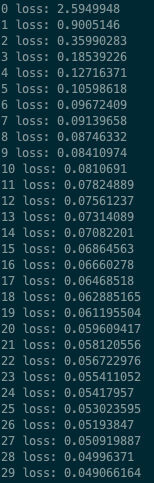print(i, “loss:”, loss.eval(feed_dict))

[Line 14] `print(i, “loss:”, loss.eval(feed_dict))`

This line prints out the loss at each epoch. On the left, you can see the value for loss is decreasing for every epoch.

The loss value is calculated using `loss.eval()` and `feed_dict` as argument.

[line 16, 17]

```print('Predicting')
y_pred_batch = session.run(y_pred, {x : x_batch})
```

After 30 epochs, we now have a trained W and b for us to perform inference. Similar to training, inference can be done with the same graph using `session.run()` but this time, the fetches will be y_pred instead of train_opand we only need to feed in x. We do this because W and b are already trained and the predicted y can be computed with just x. Notice that in`tf.add(tf.multiply(w, x), b)`, there isn’t y.

By now we have already declared 3 `session.run()`, so let’s recap their usage since `session.run()` is our command to run operations and evaluate tenors in our graph. The first time we did was to initialise our variables, second time during training to pass in our feed_dict and third time to run prediction.

[line 19–23]

```plt.scatter(x_batch, y_batch)
plt.plot(x_batch, y_pred_batch, color='red')
plt.xlim(0, 2)
plt.ylim(0, 2)
plt.savefig('plot.png')
```

We plot the chart with both the generated `x_batch` and `y_batch`, together with our predicted line (with `x_batch` and `y_pred_batch`). Finally, we have our predicted line nicely draw below. Take a moment to recap how our first neural network figures out the gradient and y-intercept, and appreciate the magic of machine learning!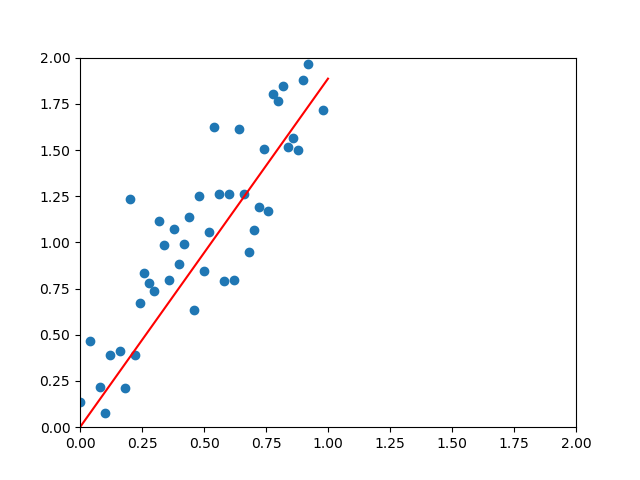plt.plot(x_batch, y_pred_batch) — we drew the line of best fit

[line 25, 56]

```if __name__ == "__main__":
run()
```

No explanation needed — you are better than this. 😉

### Lastly

Diving into machine learning is not easy. Some people start with theory, some start with code. I wrote this article to allow myself to understand the basic concept and help those who are dipping into machine learning or TensorFlow to get started.

You may find the final code here. If you spot any mistake and would like to make suggestion or improvement, please feel free to comment or tweet me. 🙏

Special thanks to RaimiRen Jie and Yuxin for reading drafts of this. You are the best! 💪

Sign up for my newsletter to get the latest update delivered to your inbox!

Last modified: July 6, 2019